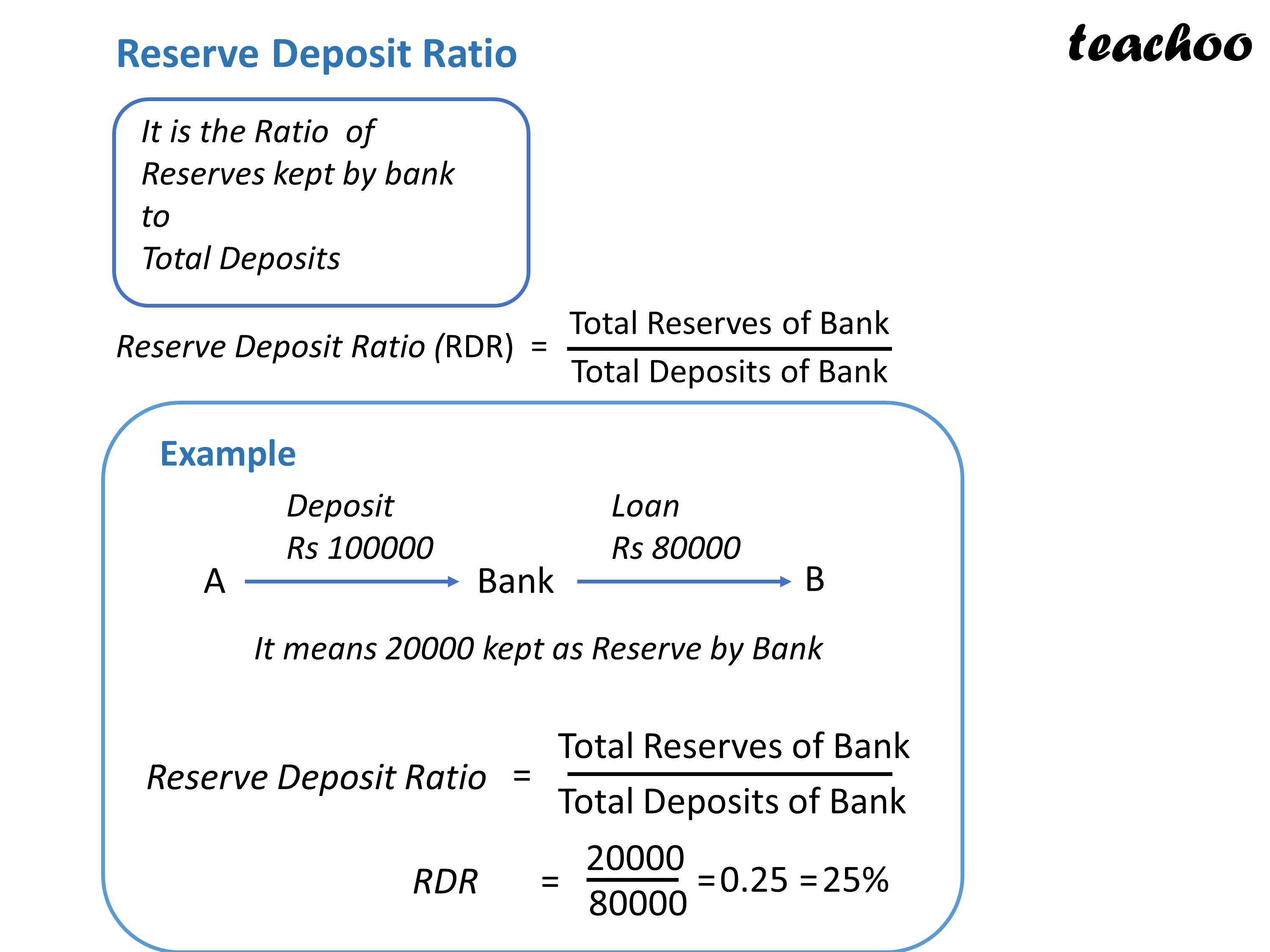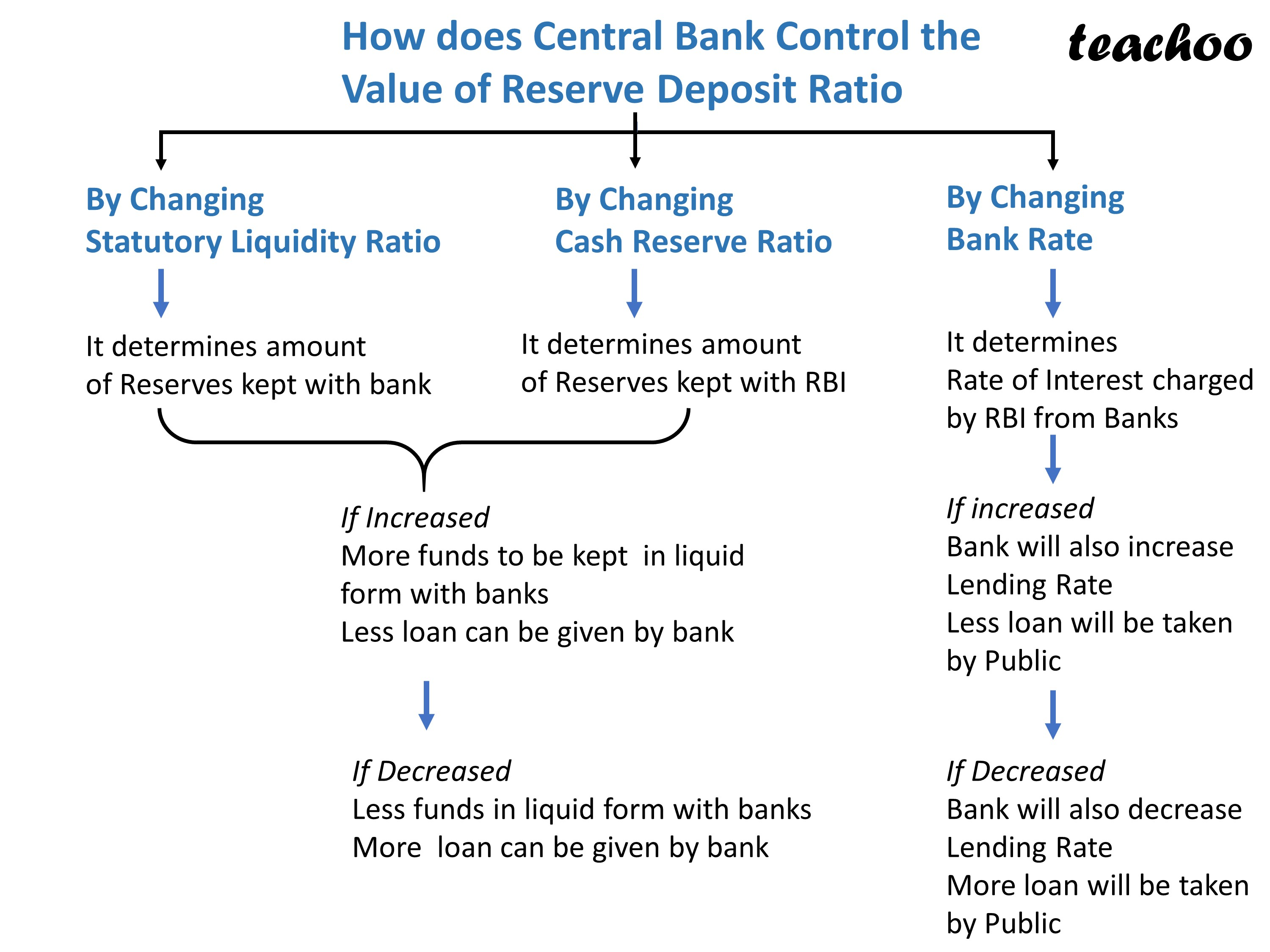Chapter 3 Part 2 - Banking

Economics Class 12
Macroeconomics

We know that

Reserve Deposit Ratio is the Ratio of Reserves kept by bank to total deposits

More the RDR,

less loan will be available to be given to bank

and vice versaThis RDR is increased or decreased by changing

Statutory liquidly Ratio

Cash Reserve Ratio

Bank Rate

as shown below#### Questions 2

Which of the following increase the money supply?

a. Fall in repo rate

b. Sale of securities in open market

c. Increase in cash reserve ratio

d. All of these

Learn in your speed, with individual attention - Teachoo Maths 1-on-1 Class

### Transcript

Reserve Deposit Ratio It is the Ratio of Reserves kept by bank to Total Deposits Reserve Deposit Ratio (RDR) Total Reserves of Bank Total Deposits of Bank Example Deposit Rs 100000 Loan Rs 80000 It means 20000 kept as Reserve by Bank Reserve Deposit Ratio Total Reserves of Bank Total Deposits of Bank RDR 80000 25% How does Central Bank Control the Value of Reserve Deposit Ratio By Changing Statutory Liquidity Ratio By Changing Cash Reserve Ratio By Changing Bank Rate It determines amount of Reserves kept with bank It determines amount of Reserves kept with RBI It determines Rate of Interest charged by RBI from Banks If Increased More funds to be kept in liquid form with banks Less loan can be given by bank If increased Bank will also increase Lending Rate Less loan will be taken by Public If Decreased Less funds in liquid form with banks More loan can be given by bank If Decreased Bank will also decrease Lending Rate More loan will be taken by Public